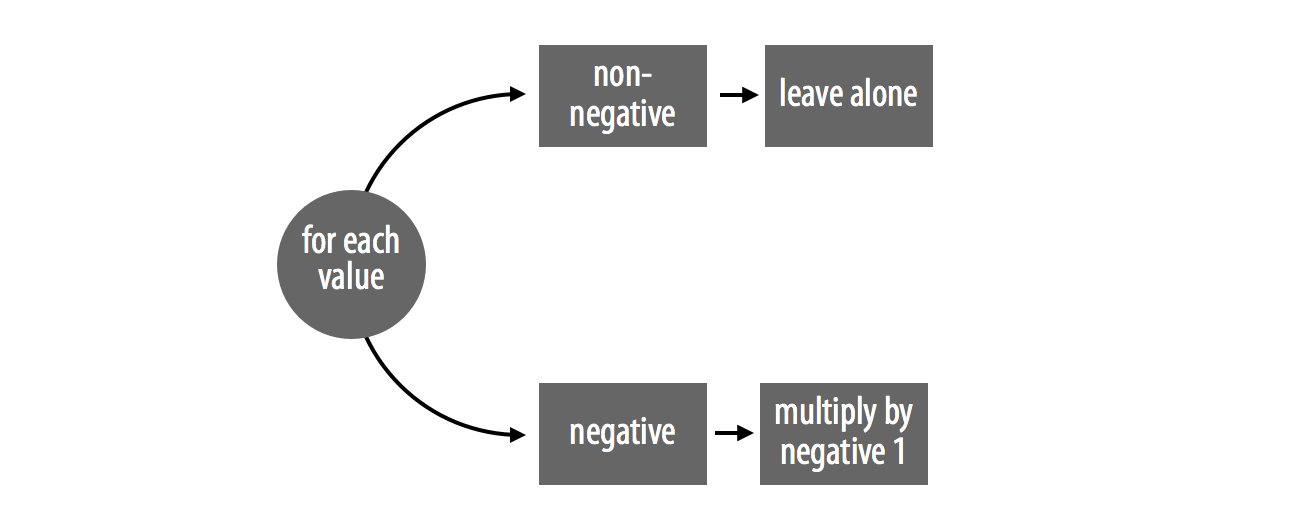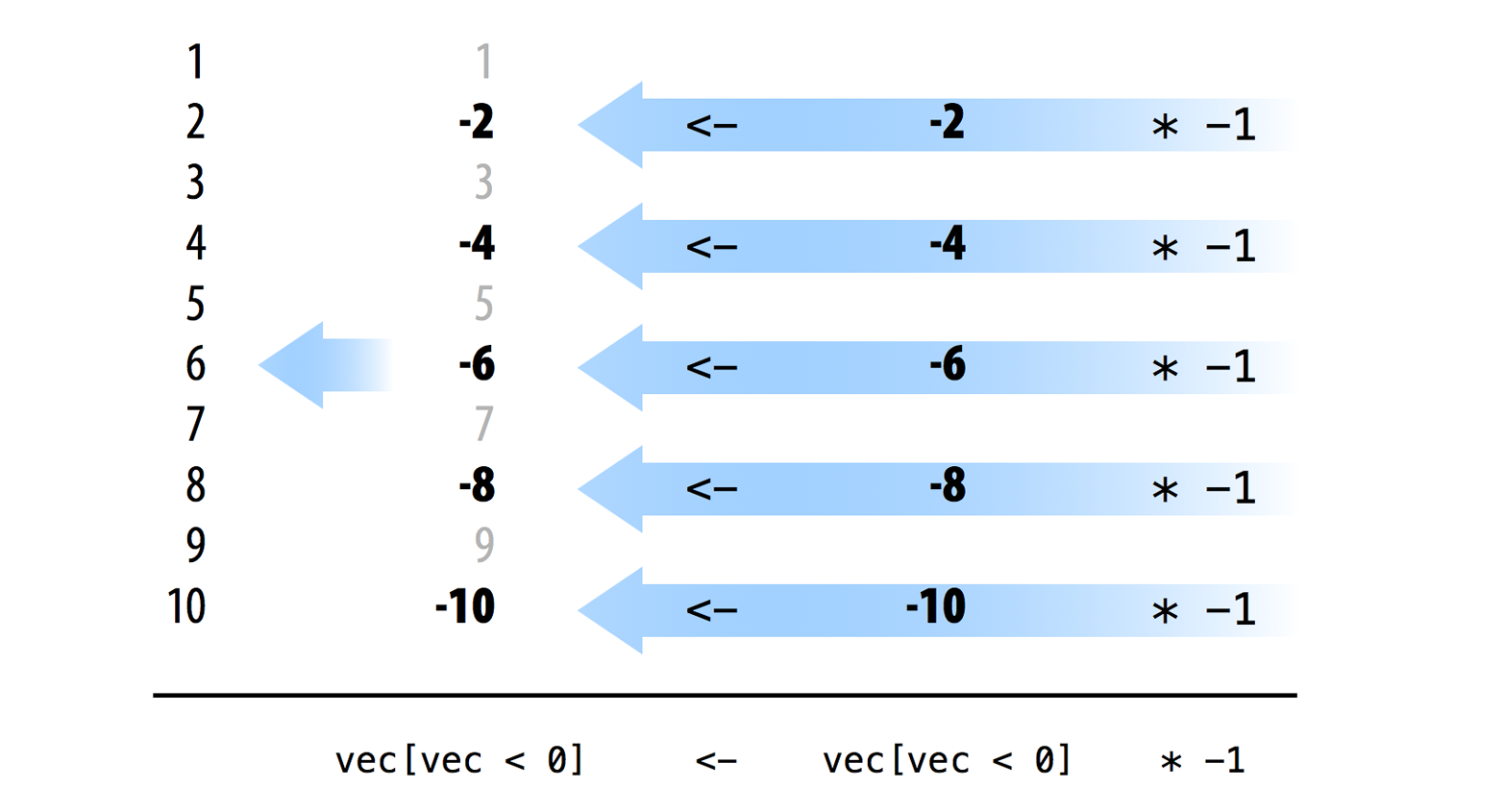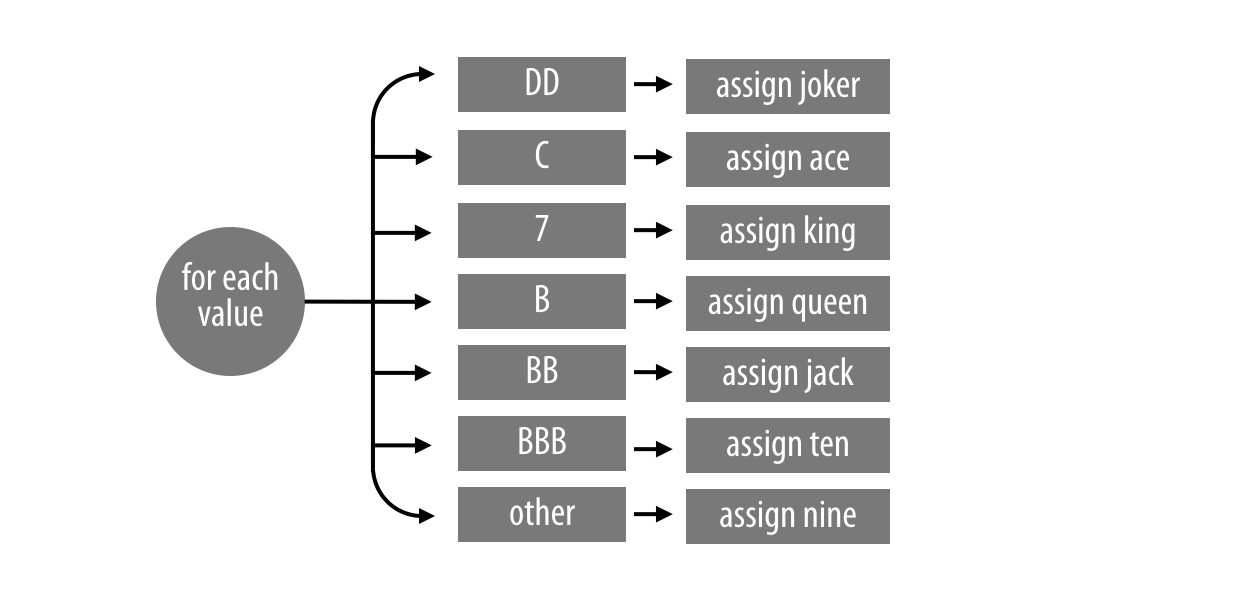# Vectorized Code

## Vectorized Code

• The fastest R code will usually take advantage of three things:
• logical tests
• subsetting
• element-wise execution
• Code that uses these things usually has a certain quality: it is vectorized.
• That means the code can take a vector of values as input and manipulate each value in the vector at the same time (parallel computing).

• Example: Write an absolute value function in R that takes
• Input: vec a vector of numbers
• Ouput: a vector with all non-negative numbers
• First method: for loop

abs_loop <- function(vec){
for (i in 1:length(vec)) {
if (vec[i] < 0) {
vec[i] <- -vec[i]
}
}
vec
}
• Second method: vectorized code
abs_vectorized <- function(vec){
negs <- vec < 0
vec[negs] <- vec[negs] * -1
vec
}
• Claim: abs_vectorized() is much faster than abs_loop().
• Let test this claim!
long <- rep(c(-1, 1), 5000000)

system.time(abs_loop(long))
##    user  system elapsed
##   0.949   0.036   0.996
system.time(abs_vectorized(long))
##    user  system elapsed
##   0.236   0.055   0.294
• How does our abs_vectorized() function compare to abs() built-in function?
system.time(abs(long))
##    user  system elapsed
##   0.028   0.001   0.029

## How to Write Vectorized Code

• To create vectorized code:
• Use vectorized functions (R built-in functions) to complete the sequential steps in your program.
• Use logical subsetting to handle parallel cases. Try to manipulate every element in a case at once.
• Example: abs_vectorized() functionvec <- c(1, -2, 3, -4, 5, -6, 7, -8, 9, -10)
vec < 0
##   FALSE  TRUE FALSE  TRUE FALSE  TRUE FALSE  TRUE FALSE  TRUE
vec[vec < 0]
##   -2  -4  -6  -8 -10
vec[vec < 0] * -1
##   2  4  6  8 10
vec
##     1  -2   3  -4   5  -6   7  -8   9 -10### Exercise 1

The following function converts a vector of slot symbols to a vector of new slot symbols. Can you vectorize it?

change_symbols <- function(vec){
for (i in 1:length(vec)){
if (vec[i] == "DD") {
vec[i] <- "joker"
} else if (vec[i] == "C") {
vec[i] <- "ace"
} else if (vec[i] == "7") {
vec[i] <- "king"
}else if (vec[i] == "B") {
vec[i] <- "queen"
} else if (vec[i] == "BB") {
vec[i] <- "jack"
} else if (vec[i] == "BBB") {
vec[i] <- "ten"
} else {
vec[i] <- "nine"
}
}
vec
}

vec <- c("DD", "C", "7", "B", "BB", "BBB", "0")

change_symbols(vec)
##  "joker" "ace"   "king"  "queen" "jack"  "ten"   "nine"Then write code that can change the symbols for each case:

vec[vec == "DD"] <- "joker"
vec[vec == "C"] <- "ace"
vec[vec == "7"] <- "king"
vec[vec == "B"] <- "queen"
vec[vec == "BB"] <- "jack"
vec[vec == "BBB"] <- "ten"
vec[vec == "0"] <- "nine"

Now, just write this into a function:

change_vec <- function (vec) {
vec[vec == "DD"] <- "joker"
vec[vec == "C"] <- "ace"
vec[vec == "7"] <- "king"
vec[vec == "B"] <- "queen"
vec[vec == "BB"] <- "jack"
vec[vec == "BBB"] <- "ten"
vec[vec == "0"] <- "nine"

vec
}

Let’s see how much faster are the vectorized code!

many <- rep(vec, 1000000)
system.time(change_symbols(many))
##    user  system elapsed
##  18.274   0.260  20.353
system.time(change_vec(many))
##    user  system elapsed
##   0.604   0.069   0.695

## Lookup Table

Another way to vectorize code is to use lookup table. Think lookup table as a dictionary.

change_vec2 <- function(vec){
tb <- c("DD" = "joker", "C" = "ace", "7" = "king", "B" = "queen",
"BB" = "jack", "BBB" = "ten", "0" = "nine")
unname(tb[vec])
}

system.time(change_vec2(many))
##    user  system elapsed
##   2.045   0.249   2.364

## How to Write Fast for Loops in R

system.time({
output <- c()

for (i in 1:1000000) {
output[i] <- i + 1
}
})
##    user  system elapsed
##   0.430   0.151   0.611
system.time({
output <- rep(NA, 1000000)

for (i in 1:1000000) {
output[i] <- i + 1
}
})
##    user  system elapsed
##   0.075   0.006   0.083

## Vectorized Code in Practice

• Remember Exercise 2 from Monday’s lecture?
• From a given list of fruits, select only the ones that have 6 or fewer letters.
fruits <- c("apple", "pineapple", "watermelon", "orange", "peach", "plum",
"honeydew", "banana", "kiwi", "papaya", "grapes", "strawberry",
"blueberry", "blackberry")

fruits_short <- c()

for (i in 1:length(fruits)) {
fruit <- fruits[i]
if (nchar(fruit) <= 6) {
fruits_short <- c(fruits_short, fruit)
}
}

fruits_short
##  "apple"  "orange" "peach"  "plum"   "banana" "kiwi"   "papaya" "grapes"
• How do we vectorize the above code?
• First, is nchar() following element-wise execution?
nchar(fruits)
##    5  9 10  6  5  4  8  6  4  6  6 10  9 10
nchar(fruits) <= 6
##    TRUE FALSE FALSE  TRUE  TRUE  TRUE FALSE  TRUE  TRUE  TRUE  TRUE FALSE
##  FALSE FALSE
fruits[nchar(fruits) <= 6]
##  "apple"  "orange" "peach"  "plum"   "banana" "kiwi"   "papaya" "grapes"

## To-do

• Submit Homework 2. Due at 11:59 PM on Compass.
• Homework 3 has been published.